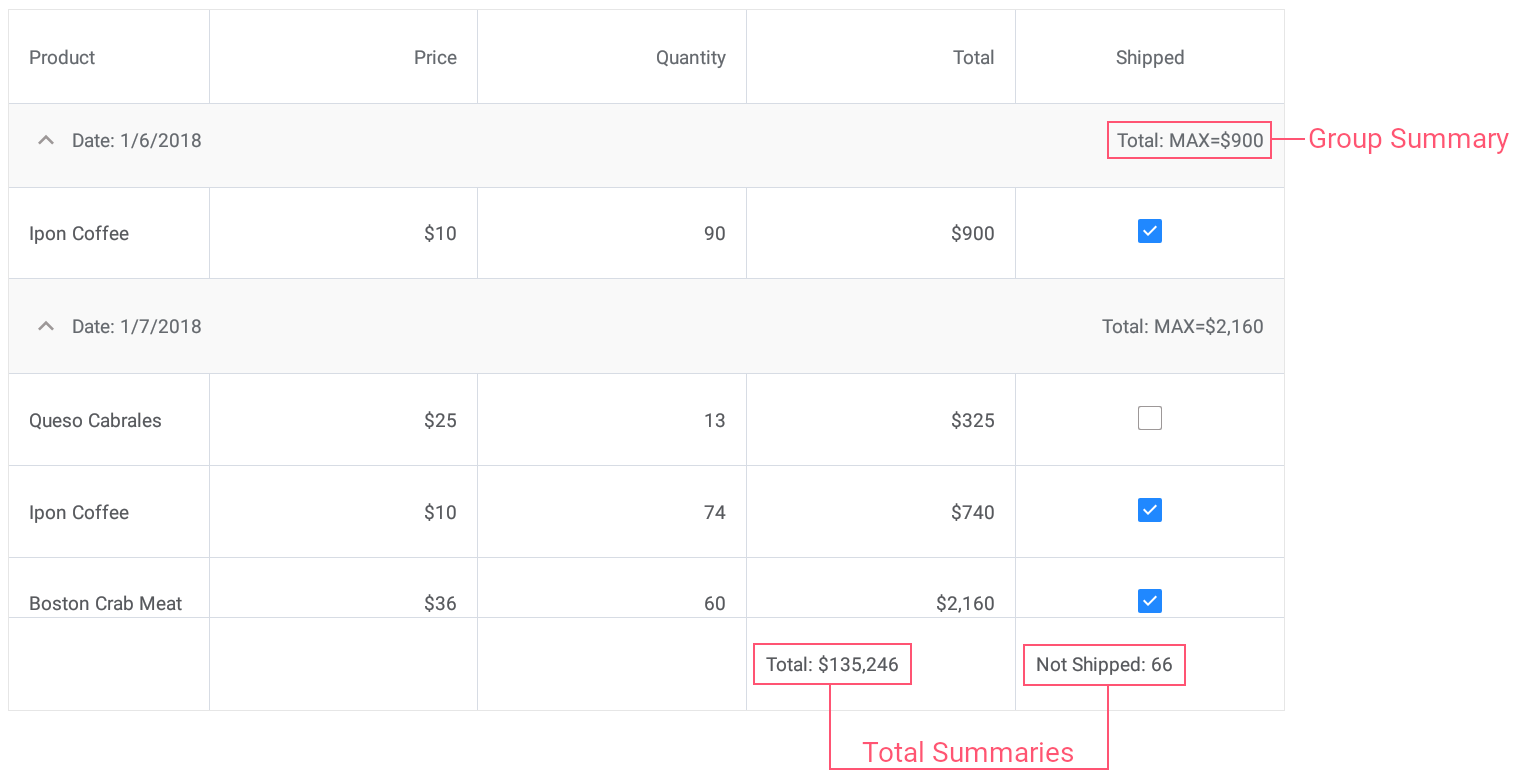# CustomSummaryEventArgs Class

Provides data for the DataGridView.CalculateCustomSummary event.

Namespace: DevExpress.XamarinForms.DataGrid

Assembly: DevExpress.XamarinForms.Grid.dll

## Declaration

``````public class CustomSummaryEventArgs :
EventArgs``````

## Remarks

The CustomSummaryEventArgs objects are automatically created, initialized and passed to the DataGridView.CalculateCustomSummary event handlers.

## Example

This example demonstrates how to use predefined aggregate functions and custom rule to calculate group and total summaries for grid columns.• Set the group summary to display the maximum Total value for each group of records.
• Set the total summary to calculate the sum of values in the Total column.
• Set the custom total summary to count the number of orders whose value in the Shipped column is false (to count orders that are not shipped).
``````<dxg:DataGridView x:Name="grid" ItemsSource="{Binding Orders}"
CalculateCustomSummary="OnCalculateCustomSummary">
<!-- ... -->
<dxg:DataGridView.GroupSummaries>
<dxg:GridColumnSummary FieldName="Total" Type="Max"/>
</dxg:DataGridView.GroupSummaries>

<dxg:DataGridView.TotalSummaries>
<dxg:GridColumnSummary FieldName="Total" Type="Sum"
DisplayFormat="Total: {0:C0}"/>
<dxg:GridColumnSummary FieldName="Shipped" Type="Custom"
DisplayFormat="Not Shipped: {0}"/>
</dxg:DataGridView.TotalSummaries>
</dxg:DataGridView>
``````
``````int count;
// ...

void OnCalculateCustomSummary(object sender, CustomSummaryEventArgs e) {
if (e.FieldName.ToString () == "Shipped")
if (e.IsTotalSummary){
if (e.SummaryProcess == CustomSummaryProcess.Start) {
count = 0;
}
if (e.SummaryProcess == CustomSummaryProcess.Calculate) {
if (!(bool)e.FieldValue)
count++;
e.TotalValue = count;
}
}
}
``````

## Inheritance

Object
EventArgs
CustomSummaryEventArgs